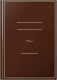# Gauss's LawFrederic P. Miller, Agnes F. Vandome, John McBrewster
VDM Publishing, 2009 - 74 sivua
In physics, Gauss's law, also known as Gauss's flux theorem, is a law relating the distribution of electric charge to the resulting electric field. Gauss's law states that: The electric flux through any closed surface is proportional to the enclosed electric charge. The law was formulated by Carl Friedrich Gauss in 1835, but was not published until 1867. It is one of the four Maxwell's equations, which form the basis of classical electrodynamics. Gauss's law can be used to derive Coulomb's law, and vice versa. The integral and differential forms are related by the divergence theorem, also called Gauss's theorem. Each of these forms can also be expressed two ways: In terms of a relation between the electric field E and the total electric charge, or in terms of the electric displacement field D and the free electric charge. Gauss's law has a close mathematical similarity with a number of laws in other areas of physics. See, for example, Gauss's law for magnetism and Gauss's law for gravity.

### Mitä ihmiset sanovat -Kirjoita arvostelu

Yhtään arvostelua ei löytynyt.